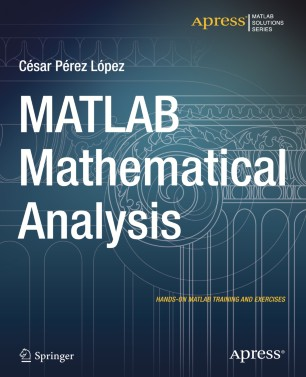# MATLAB Mathematical Analysis

• AuthorsBook

1. Front Matter
Pages i-xxv
2. César Pérez López
Pages 1-12
3. César Pérez López
Pages 13-70
4. César Pérez López
Pages 71-124
5. César Pérez López
Pages 125-182
6. César Pérez López
Pages 183-202
7. César Pérez López
Pages 203-224
8. César Pérez López
Pages 225-278
9. César Pérez López
Pages 279-324
10. César Pérez López
Pages 325-346

### Introduction

MATLAB Mathematical Analysis is a reference book that presents the techniques of mathematical analysis through examples and exercises resolved with MATLAB software. The purpose is to give you examples of the mathematical analysis functions offered by MATLAB so that you can use them in your daily work regardless of the application. The book supposes proper training in the mathematics and so presents the basic knowledge required to be able to use MATLAB for calculational or symbolic solutions to your problems for a vast amount of MATLAB functions.

The book begins by introducing the reader to the use of numbers, operators, variables and functions in the MATLAB environment. Then it delves into working with complex variables. A large section is devoted to working with and developing graphical representations of curves, surfaces and volumes. MATLAB functions allow working with two-dimensional and three-dimensional graphics, statistical graphs, curves and surfaces in explicit, implicit, parametric and polar coordinates. Additional work implements twisted curves, surfaces, meshes, contours, volumes and graphical interpolation.

The following part covers limits, functions, continuity and numerical and power series. Then differentiation is addressed in one and several variables including differential theorems for vector fields. Thereafter the topic of integration is handled including improper integrals, definite and indefinite integration, integration in multiple variables and multiple integrals and their applications.

Differential equations are exemplified in detail, Laplace transforms, Tayor series, and the Runga-Kutta method and partial differential equations.